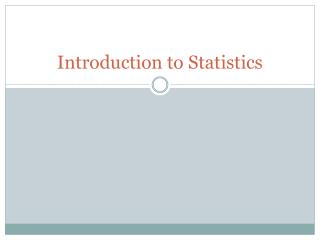# Introduction to Statistics - PowerPoint PPT PresentationDownload PresentationIntroduction to Statistics

Introduction to Statistics
Download Presentation## Introduction to Statistics

- - - - - - - - - - - - - - - - - - - - - - - - - - - E N D - - - - - - - - - - - - - - - - - - - - - - - - - - -
##### Presentation Transcript

1. Introduction to Statistics

2. Introduction to Statistics • Statistics is the Science of data • Any set of data contains information about some group of individuals. The information is organized in variables • Individuals are the objects described by a set of data. (they can be people, animals, or things) • A variable is any characteristic of an individual.

3. Types of Variables Categorical – places the individual into a group Quantitative – take a numerical value

4. Exploring Data • Example: for the set of data answer the following – • What Individuals do the data describe? • Adults • How many variables are there? What are they? • Three: Marital status, Count, and Percent • In what units is each variable recorded? • Count is recorded by the million, and Percent • Are the variables quantitative or categorical? • Marital Status is categorical and Count and Percent are both quantitative